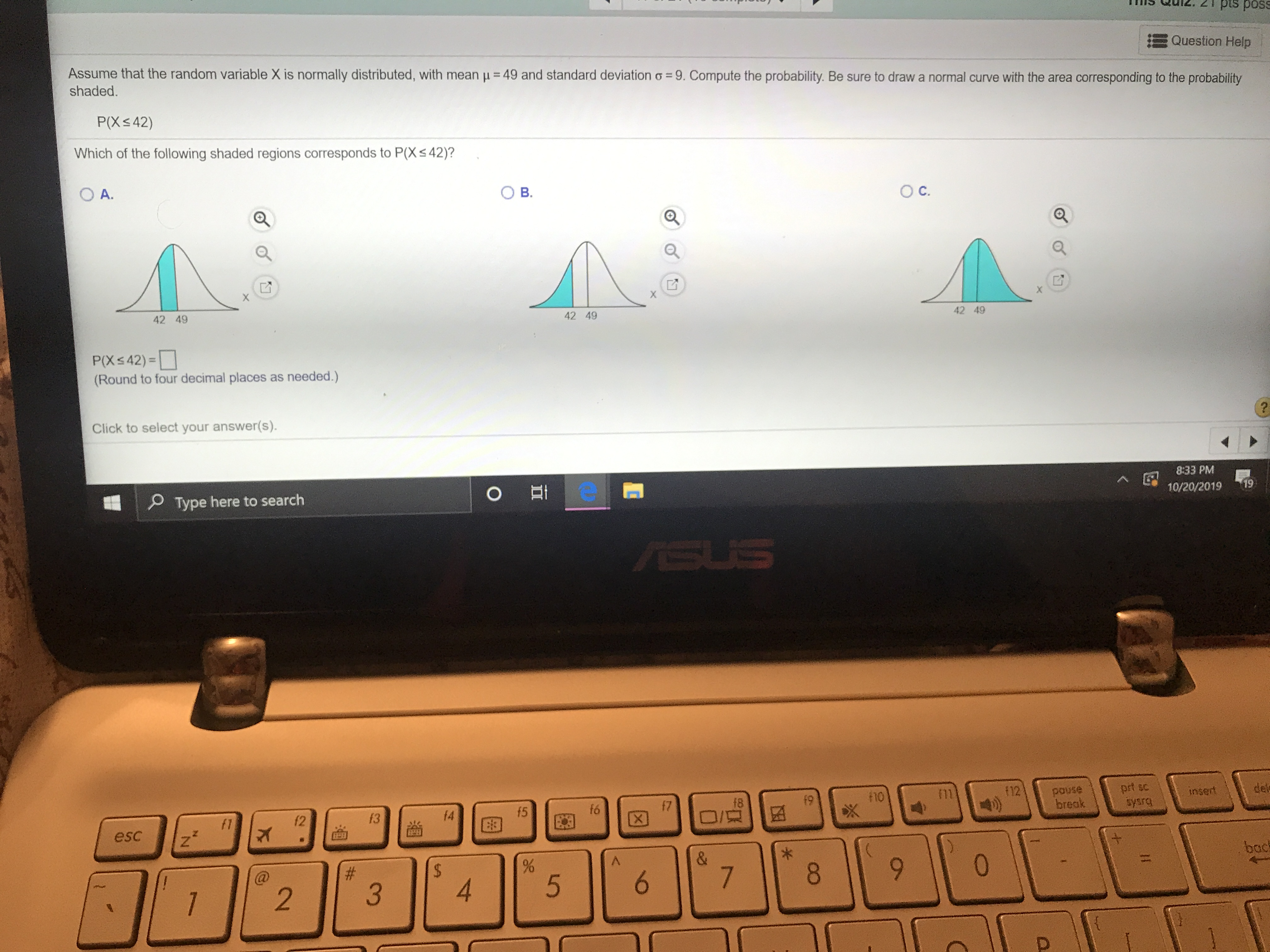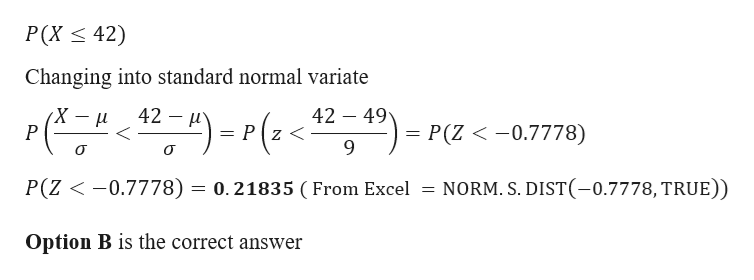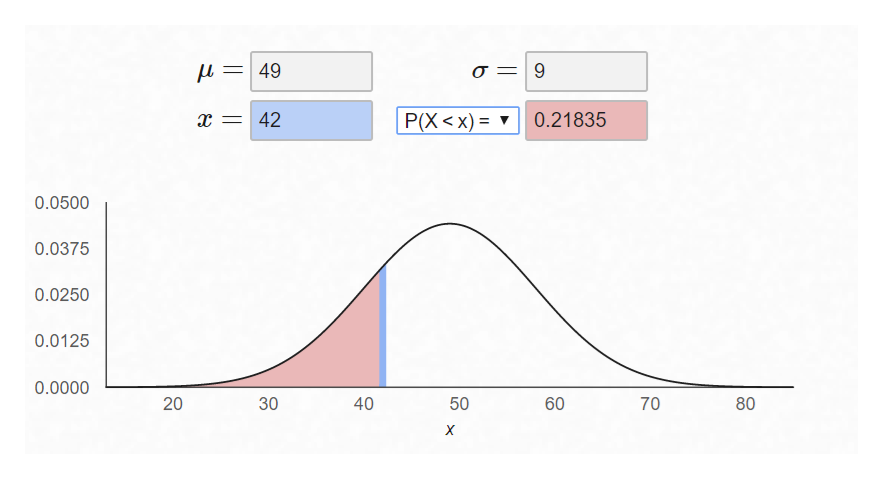# d..SSod sid z 7inn sutQuestion HelpAssume that the random variable X is normally distributed, with mean u 49 and standard deviation o 9. Compute the probability. Be sure to draw a normal curve with the area corresponding to the probabilityshaded.P(X< 42)Which of the following shaded regions corresponds to P(Xs42)?O C.O A.42 4942 4942 49P(X

Question

Helphelp_outlineImage Transcriptionclosed.. SSod sid z 7inn sut Question Help Assume that the random variable X is normally distributed, with mean u 49 and standard deviation o 9. Compute the probability. Be sure to draw a normal curve with the area corresponding to the probability shaded. P(X< 42) Which of the following shaded regions corresponds to P(Xs42)? O C. O A. 42 49 42 49 42 49 P(X<42)= (Round to four decimal places as needed.) Click to select your answer(s) 8:33 PM 10/20/2019 61 Type here to search E O prt sc f12 snod break insert f8 61 15 91 12 /O esc % 0 \$ # 9 OO fullscreen
check_circleExpert Solution
Step 1

Given data
Mean = 49
Standard deviation = 9help_outlineImage TranscriptioncloseР(X < 42) Changing into standard normal variate 42 49 42 (X- P(Z 0.7778) Pz< 9 Р P(Z 0.7778) = 0.21835 ( From Excel NORM. S. DIST(-0.7778, TRUE)) Option B is the correct answer fullscreen
Step 2

Graph has been sh...help_outlineImage Transcriptionclose=49 9 P(X < x) X 42 0.21835 0.0500 0.0375 0.0250 0.0125 0.0000 40 70 80 X 60 50 30 20 fullscreen

### Want to see the full answer?

See Solution

#### Want to see this answer and more?

Solutions are written by subject experts who are available 24/7. Questions are typically answered within 1 hour*

See Solution
*Response times may vary by subject and question
Tagged in

### Other